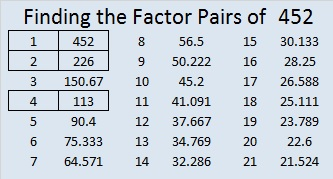# 452 and Level 4

52 is divisible by 4 because 2 is an even number that is not divisible by 4, and the digit before it, 5, is an odd number. Thus 452 and ANY OTHER number ending in 52 is divisible by 4.

Here is another divisibility trick: 1% of all numbers end in the digits 52, which is NOT divisible by 8.  No matter how long the number is, if the digit immediately preceding those ending digits of 52 is odd, then that number will be divisible by 8, and if that digit is even, the number will NOT be divisible by 8. Thus 452 is NOT divisible by 8.

Live, Love, Laugh recently wrote a post about the Find the Factors blog. Check them out.

This Level 4 puzzle is a little tougher than usual, but if you’ve done other Level 4 puzzles, I think you can still handle it.Print the puzzles or type the factors on this excel file:  12 Factors 2015-04-06

——————————————————————————————————————-

• 452 is a composite number.
• Prime factorization: 452 = 2 x 2 x 113, which can be written 452 = (2^2) x 113
• The exponents in the prime factorization are 2 and 1. Adding one to each and multiplying we get (2 + 1)(1 + 1) = 3 x 2  = 6. Therefore 452 has exactly 6 factors.
• Factors of 452: 1, 2, 4, 113, 226, 452
• Factor pairs: 452 = 1 x 452, 2 x 226, or 4 x 113
• Taking the factor pair with the largest square number factor, we get √452 = (√4)(√113) = 2√113 ≈ 21.2603Since 452 has only 6 factors, we would get a two-layer cake when we use the cake method to find its factors. Dividing 452 by 4 is easier than dividing it by 2 twice, so I’ve modified the cake method to get just a one-layer cake to find the square root of 452. Since 113 is a prime number, no other divisions are possible.To simplify the square root of 452, simply take the square root of every number on the outside of the cake. Thus √452 = (√4)(√113) = 2√113.

——————————————————————————————————————-## 2 thoughts on “452 and Level 4”

1.Paula Beardell Krieg

Did you see the well deserved nod to your puzzles on Jo Morgan’s Resourceaholic Gem post? She wrote

“Some things are hard to categorise, but I spot them on Twitter and they capture my imagination. The winner of this category is @IvaSallay’s Find the Factors resources. I featured these in Gems 11 – they’re ‘an excellent way for children and adults to review multiplication facts, use logic, and strengthen brain power’. They are lovely puzzles”.
http://www.resourceaholic.com/2015/04/gem-awards-2015.html

Jo has good taste. 🙂

•ivasallay

Your comment was one of the first things I saw when I came home from work. Thanks for letting me know!

This site uses Akismet to reduce spam. Learn how your comment data is processed.